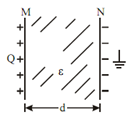## Capacitance between two Parallel Plates Assignment Help

Assignment Help: >> Capacitance - Capacitance between two Parallel Plates

Capacitance between Two Parallel Plates:

A parallel plate capacitor contain two plates M and N each of area A m2 separated by thickness d metres of medium of relative permittivity εr. If charge + Q coulomb is given to plate M, then flux passing out through medium is ψ = Q

Flux density in medium, D = ψ / A = Q / A.Figure: Capacitor with Dielectric

E = V /d           and         D = ε E

or         Q/ A =ε V /d

∴          Q/ V = A ε/ d

∴          C = (ε0 εr A) /d   farad (in a medium of permittivity, ε = ε0 εr) and

C = ε0 A /d      farad (in air as medium)

 Capacitance in Series and Parallel Energy Stored in a Capacitor